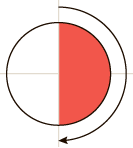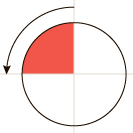CSS <angle> Data Type

Description

The <angle> CSS data type sets the angle of inclination or rotation. A positive value is measured clockwise, a negative value counter clockwise. The <angle> consists of a <number> followed by either deg, rad, grad or turn. The number is immediately followed by the unit, no white space is allowed between them, and the length units are case-insensitive, i.e. 90deg and 90DEG are treated the same.

Syntax

property: <angle>;

Values

• degRepresents an angle in degrees. There are 360 degrees in a full circle.
• turnRepresents an angle in a number of turns. There is 1 turn in a full circle.

The values ​​of some angles are presented in the table below:90deg = 100grad = 0.25turn ≈ 1.5708rad180deg = 200grad = 0.5turn ≈ 3.1416rad270deg = 300grad = 0.75turn ≈ 4.7124rad-90deg = -100grad = -0.25turn ≈ -1.5708rad

Example

<p>Example of the &lt;angle&gt; css data type using  gradients.</p>
body {
background: #CE5937;
background: -moz-linear-gradient(-45deg, #CE5937 0%, #1C6EA4 50%, #C59237 100%);
background: -webkit-linear-gradient(-45deg, #CE5937 0%, #1C6EA4 50%, #C59237 100%);
background: linear-gradient(135deg, #CE5937 0%, #1C6EA4 50%, #C59237 100%);
}
p {
background: #fff;
 Desktop9 12 2 3.6 15 4 Tablets / Mobile2 18 4 14 3.2 1.0